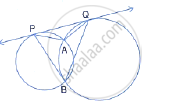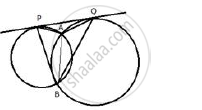Share

# Two Circles Intersect Each Other at Points a and B. Their Common Tangent Touches the Circles at Points P and Q as Shown in the Figure. Show that the Angles Paq and Pbq Are Supplementary. - Mathematics

Course

#### Question

Two circles intersect each other at points A and B. their common tangent touches the circles at points P and Q as shown in the figure. Show that the angles PAQ and PBQ are supplementary.#### SolutionJoin AB.
PQ is the tangent and AB is a chord
∴ ∠QPA = ∠PBA …………(i) (angles in alternate segment)
Similarly,
∠PQA  = ∠QBA ………… (ii)

∠ QPA + ∠PQA  = ∠PBA + ∠QBA
But, in Δ PAQ,
∠QPA + ∠PQA = 180°  - ∠PAQ …… (iii)
And ∠PBA+ ∠QBA = ∠PBQ ……..(iv)
From (iii) and (iv)
∠PBQ  = 180°  - ∠PAQ
⇒ ∠PBQ + ∠PAQ = 180°
⇒ ∠PBQ + ∠PBQ  = 180°
Hence ∠PAQ and ∠PBQ are supplementary

Is there an error in this question or solution?